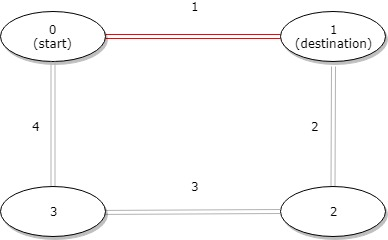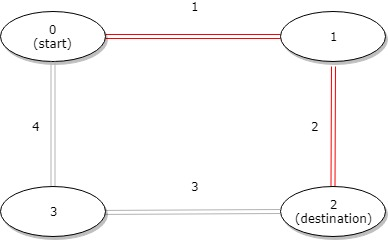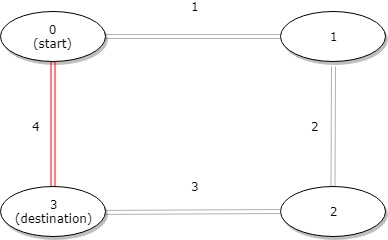1184. Distance Between Bus Stops

# 1184. Distance Between Bus Stops#

## 题目 #

A bus has `n` stops numbered from `0` to `n - 1` that form a circle. We know the distance between all pairs of neighboring stops where `distance[i]` is the distance between the stops number `i` and `(i + 1) % n`.

The bus goes along both directions i.e. clockwise and counterclockwise.

Return the shortest distance between the given `start` and `destination` stops.

Example 1:``````Input: distance = [1,2,3,4], start = 0, destination = 1
Output: 1
Explanation: Distance between 0 and 1 is 1 or 9, minimum is 1.
``````

Example 2:``````Input: distance = [1,2,3,4], start = 0, destination = 2
Output: 3
Explanation: Distance between 0 and 2 is 3 or 7, minimum is 3.
``````

Example 3:``````Input: distance = [1,2,3,4], start = 0, destination = 3
Output: 4
Explanation: Distance between 0 and 3 is 6 or 4, minimum is 4.
``````

Constraints:

• `1 <= n <= 10^4`
• `distance.length == n`
• `0 <= start, destination < n`
• `0 <= distance[i] <= 10^4`

## 题目大意 #

• 1 <= n <= 10^4
• distance.length == n
• 0 <= start, destination < n
• 0 <= distance[i] <= 10^4

## 解题思路 #

• 给出一个数组，代表的是公交车站每站直接的距离。距离是按照数组下标的顺序给出的，公交车可以按照顺时针行驶，也可以按照逆时针行驶。问行驶的最短距离是多少。
• 按照题意，分别算出顺时针和逆时针的行驶距离，比较两者距离，取出小值就是结果。

## 代码 #

``````
package leetcode

func distanceBetweenBusStops(distance []int, start int, destination int) int {
clockwiseDis, counterclockwiseDis, n := 0, 0, len(distance)
for i := start; i != destination; i = (i + 1) % n {
clockwiseDis += distance[i]
}
for i := destination; i != start; i = (i + 1) % n {
counterclockwiseDis += distance[i]
}
if clockwiseDis < counterclockwiseDis {
return clockwiseDis
}
return counterclockwiseDis
}

``````Apr 8, 2023Edit this page